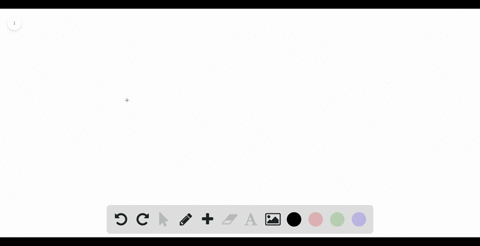### Use Definition 2 to find an expression for the ar…

00:53University of California, Berkeley
Problem 22

Use Definition 2 to find an expression for the area under the graph of $f$ as a limit. Do not evaluate the limit.

$f(x) = x^2 + \sqrt{1 + 2x}, \hspace{5mm} 4 \le x \le 7$

Area $=\lim _{n \rightarrow \infty} \frac{3}{n} \sum_{i=1}^{n}\left[\left(4+\frac{3 i}{n}\right)^{2}+\sqrt{9+\frac{6 i}{n}}\right]$

## Discussion

You must be signed in to discuss.

## Video Transcript

Okay, The first thing we know is that a is for and be a seven. Therefore, we know that Delta X is gonna be seven minus for divide by ad, which is equivalent to three over and therefore the area is the limit as an approaches infinity.